GROWTH OF NUMBERS (as Holons, each with specific layers).
The REAL PASCAL TRIANGLE (with hidden information)

Next two images show the causal diagram of how the membrane can couple locally in unique sub-sets. The second image is dynamic.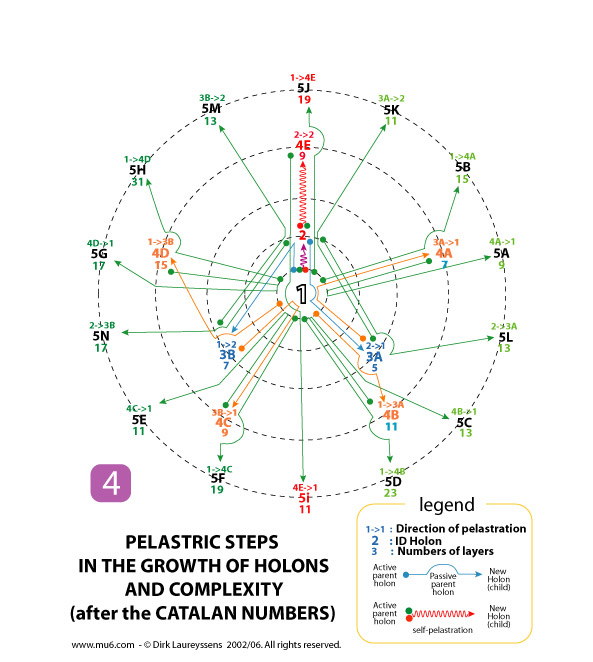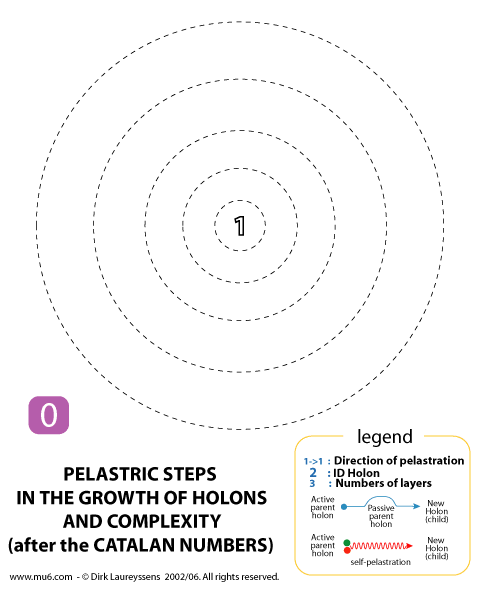You can see in next Pascal Triangle that each Catalan number is the sum of specific Pascal numbers. The Catalan numbers occupy all Pascal numbers except the middle row and the rows of ones.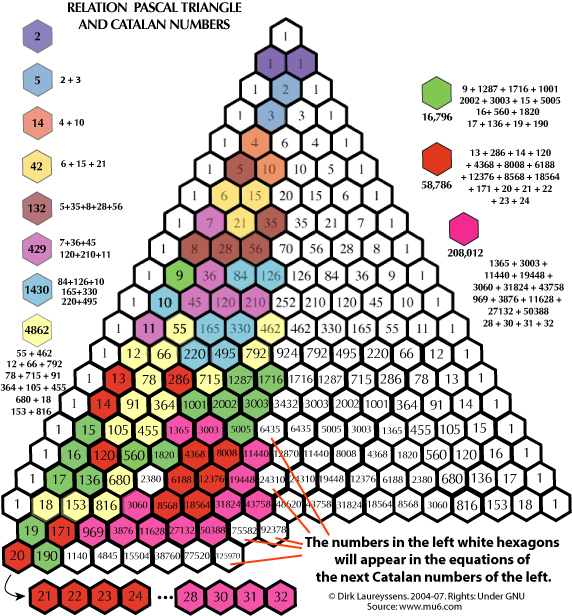What is the logic behind the difference in layering? The direction of a pelastration (membrane penetration) creates different membrane combinations in holons. Which holon is active and which is passive determinates the number of layers. You can check this in next image which shows only two steps of coupling. On the right the holon 2 pelastrates a new part of the membrane, creating that way the (child) holon 3A with 5 layers. On the left the holon 2 is pelastrated by a part of the membrane, creating that way the (child) holon 3B which has 7 layers.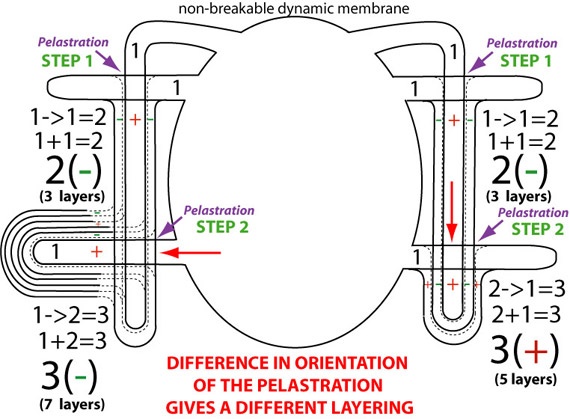When we combine this new information we can state that till now we had a wrong idea about the real Pascal Triangle. From the understanding that there is one background field (which Tesla called: The Field of Force) that we call the membrane, we say that there is infinite neutral energy, called the Zero Field Energy. Puthoff calls it wrongly the Zero Point Energy. The zero field energy (ZFE) can create locally holons. These holons can be seen as abstract units in mathematical sense, also energetically as entangled dynamic forces, also as spaces in cosmology and as fundamental particles. When we put this general idea in a diagram which will relate to the Pascal's Triangle we get this images: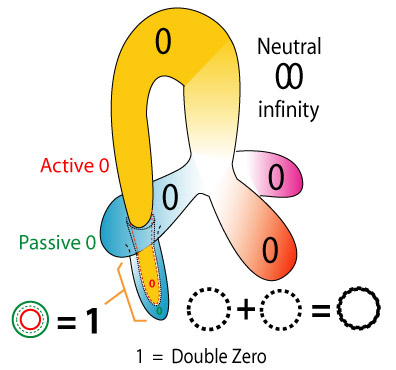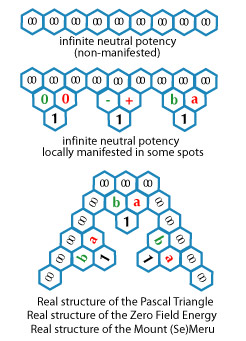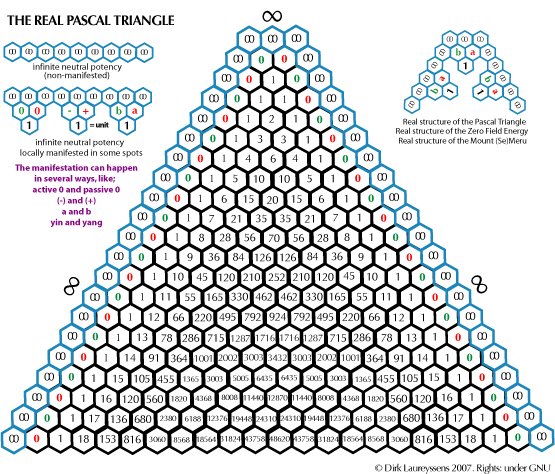This external addition to the Pascal's Triangle gives us new information about the hidden structure of the triangle. The traditional Pascal's Triangle is in fact compressed. Between each "one" there should be at least one empty zone, which represents the isolation between each "unit". This is clear in next "mathematical analysis" of the Pascal Triangle.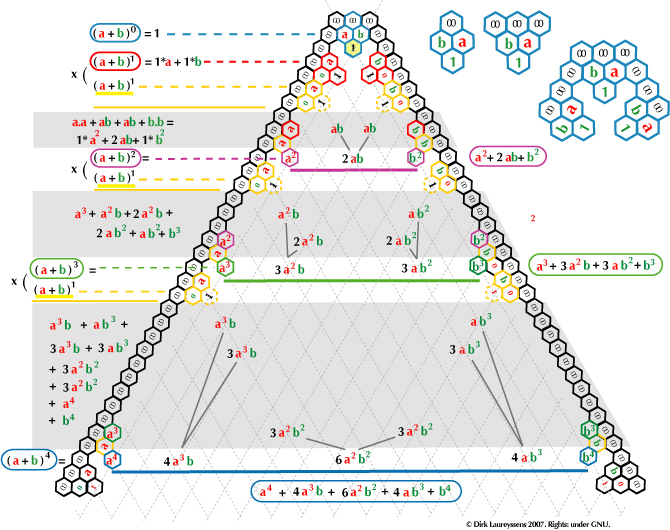In above image of the real Pascal Triangle I showed how two joined zero's - having together three layers following the topological logic - will create the number One. The number One is thus a Union, acting or observed as a Unit (1).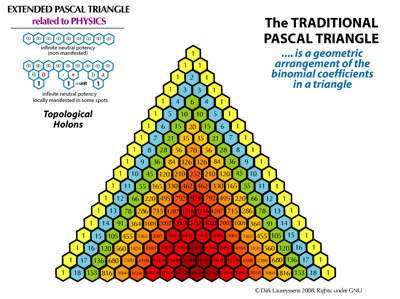Above animated image of the ZERO PASCAL TRIANGLE related to physics shows how we can explain the strange concept of the so called "zero point energy" by a single Zero Field - a membrane - that represents the "pure energy" where Frank Wilczek refers to in his new book. A larger animated image or .mov movie can be found here.

When we look to the traditional approach related to the Fibonacci Numbers we see this block diagram on wikipedia: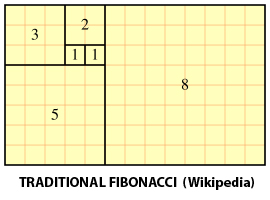The first One that you see here is however a enigma in mathematics. What is 1^0? There are different opinions between mathematicians. In the Pelastratic approach this enigmatic first One is composed by two zero's (two background membrane peaks), making a new union that has three layers. That gives next picture:ONE is a topological union of two zero's where one zero is active - the penetrator - and the other one is a passive peak tube. The passive and active peak have each another origin, here represented by an opposite spiral motion. Since the topological penetration (pelastration) creates three membranes in this union a "space" - with three dimensions - - is created. Now the image of two separating curves may remember you to images of particle collisions in particle colliders like at Cern. See next images about the decay of some fundamental particles.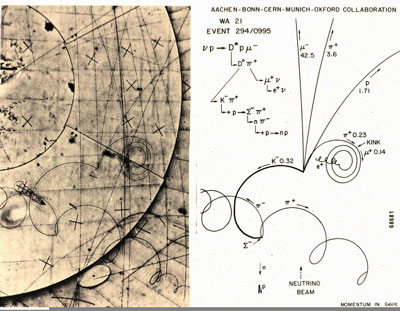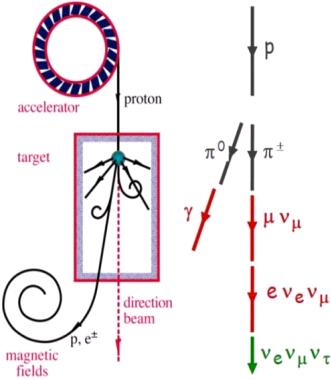We see in those three images how fundamental particles - being UNITS - will decay into other units, proving that they are Unions, similar how the number One is composed by at least two parts (zero's). It's that what the Casimir effect, virtual particles and vacuum energy (Zero Field/point Energy) are about? And what binary expressions (zero's and one's) are about? A One (1) in electronics means that curvature of "space-time" envelops or enfolds neutral energy in a measurable "shape".
In mathematical sense this approach means in the Fibonacci square diagram that the first square of One (1^0) is in fact composed by (vacuum) zero's. That gives a completely new type of Fibonacci diagram that find it's confirmation in the reality of particle Physics (like in Cern Experiments):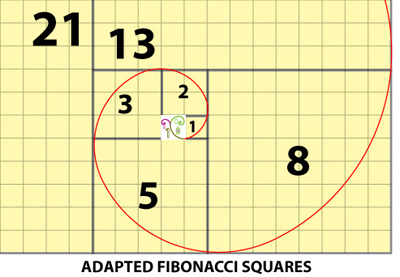Indeed the first One didn't come out of the Quantum Magic Blue but has a logic topological and geometrical origin, like Riemann, Clifford and Einstein ("God doesn't play dice") tried to make us understand.
This approach is comform with the geometric concept that
matter and energy are simply different types of curvature of space.
Influenced by the non-Euclidean geometries of Bernhard Riemann and Nikolay Lobachevsky, William Kingdon Clifford presented in 1876 such idea. He was thus preambular to Albert Einstein's General Relativity theory, who called this "deformations" of spacetime or topological defects. So Riemann Riemann showed in 1854 - based on a crumpled paper ball - that as there are different kinds of lines and surfaces, so there are different kinds of space of three dimensions, including hidden dimensions.